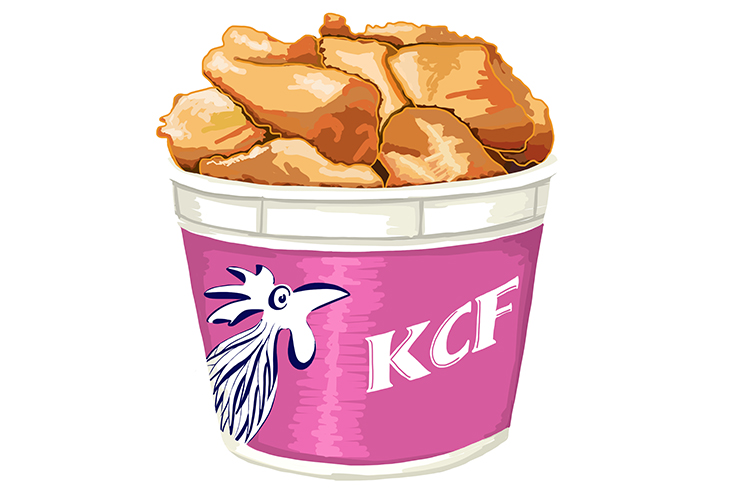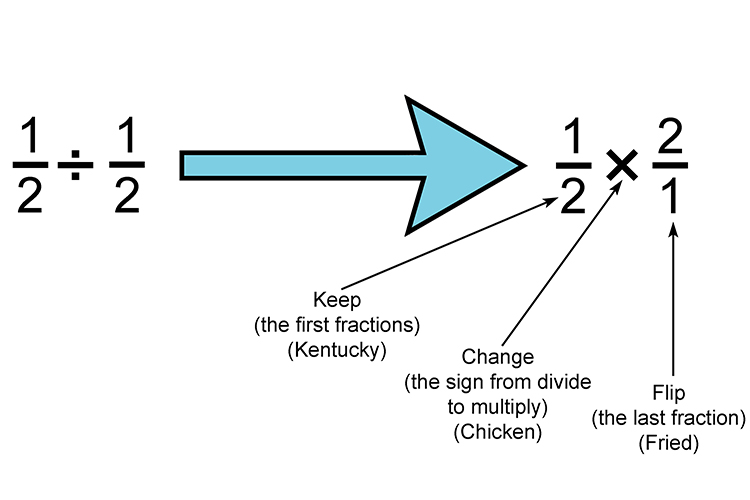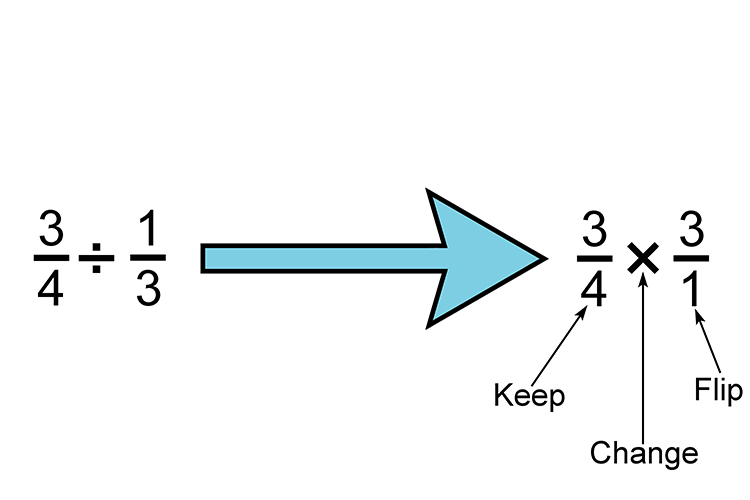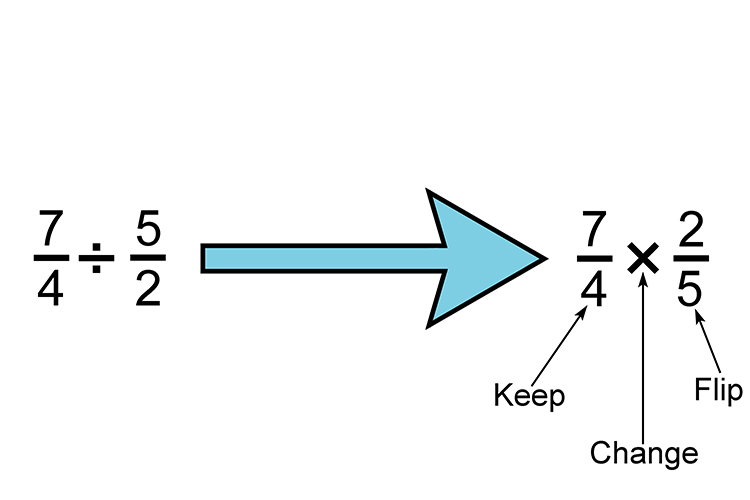# DIVIDING FRACTIONS THINK KENTUCKY CHICKEN FRIEDShouldn’t it be KFC? No it’s swapped around.

Notice the name is switched a little from that most famous fast chicken joint to KENTUCKY CHICKEN FRIED.

K – Keep (the first fraction)

C – Change (the sign from divide to multiply)

F – Flip (the last fraction)

Example 1And from multiplication of fractions we know that

1/2 \times2/1=(1times2)/(2times1)=2/2=1

Example 2

Work out  3/4\div1/3And from multiplication of a fraction we know that

3/4\times3/1=(3\times3)/(4times1)=9/4=2\1/4

Example 3

Work out  1\3/4\div2\1/2

This means work out  7/4\div5/2And from multiplication of a fraction we know that

7/4\times2/5=(7times2)/(4times5)=14/20=7/10